0

# Custom Murals Wallpaper Modern 3D Stereo Abstract Art

Custom Murals Wallpaper Modern 3D Stereo Abstract Art Geometric Photo Wall Painting Living Room Bedroom Background Wall Covering

◆Price :\$ / Square meter(The website price is only for 1 square meter)

How to place the order

Step 1:  Measure your wall dimension: Height * Width  = Square meter

Example: You wall size : Width = 3.2 meters ; Height = 2.8 meters.

According to area size is: 3.2 M * 2.8 M = 8.96 Square meters.

Step 2:   Choose the right quantity in the order.  Square meter = Order Quantity

If you need 9 square meters, Please choose quantity 9 in the order.

If you need 7.1- 7.9 square meter, Please place the order of 8 square meter.

Price = 1 square meter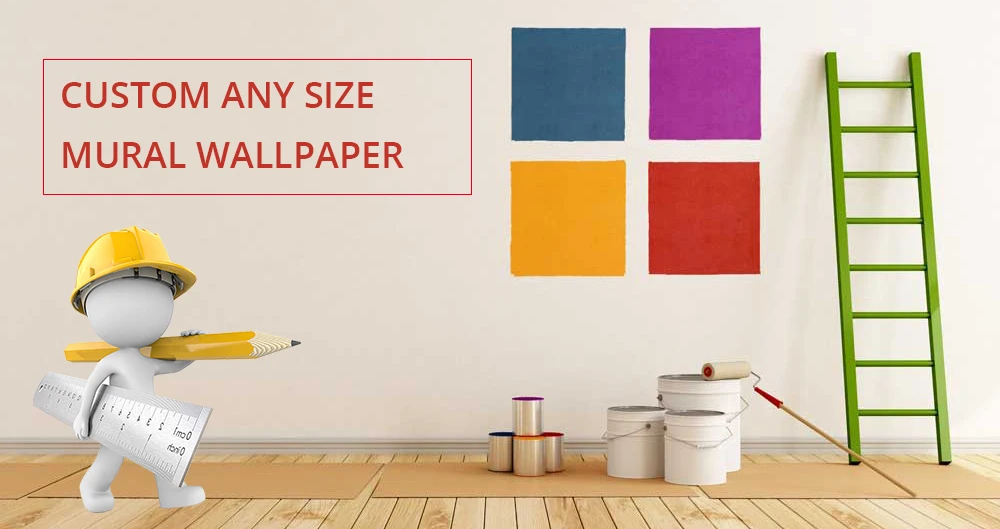Custom Mural Wallpaper

Material Feature : Self-Adhesive / Waterproof / Strong Stickiness / Removable

Price :\$ / Square meter(The website price is only for 1 square meter)

Use : Living room / Bedroom / Wedding House / Kid's Room / Study / Bathroom

Size : Customized size (Tell us Width & Height)

Default Size :

1 square meter=140cm(W) x 70cm(H) (4'7" x 2'4") ft
2 square meter=200cm(W) x 100cm(H) (6'7" x 3'3") ft
3 square meter=220cm(W) x 140cm(H) (7'3" x 4'7") ft
4 square meter=250cm(W) x 160cm(H) (8'2" x 5'3") ft
5 square meter=280cm(W) x 180cm(H) (9'2" x 5'11") ft
6 square meter=300cm(W) x 200cm(H) (9'10" x 6'7") ft
7 square meter=330cm(W) x 210cm(H) (10'10" x 6'11") ft
8 square meter=360cm(W) x 230cm(H) (11'10" x 7'6") ft
9 square meter=380cm(W) x 240cm(H) (12'5" x 7'10") ft
10 square meter=400cm(W) x 250cm(H) (13'1" x 8'2") ft
11 square meter=420cm(W) x 260cm(H) (13'9'' x 8'6'') ft
12 square meter=440cm(W) x 270cm(H) (14'5" x 8'10") ft
13 square meter=460cm(W) x 280cm(H) (15'1'' x 9'2'') ft
14 square meter=480cm(W) x 290cm(H) (15'9'' x 9'6'') ft
15 square meter=500cm(W) x 300cm(H) (16'5'' x 9'10'') ft
16 square meter=500cm(W) x 320cm(H) (16'4" x 10'5") ft

FAQ 1
: I need 1 set of mural wallpaper, the size is 2.8m width and 2.6m height. How to order it ?
Area = width x height.   Square meters = Order Quantity
Area = 2.8m x 2.6m= 7.28m2, So you should order 8 square meters. Please choose quantity 8 in the order.

If you need 8 square meters, please choose "Material" "1 m²" and "Quantity 8" here.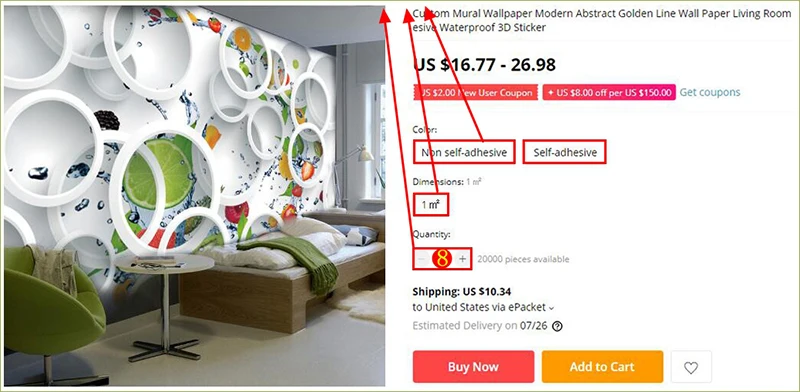Product Show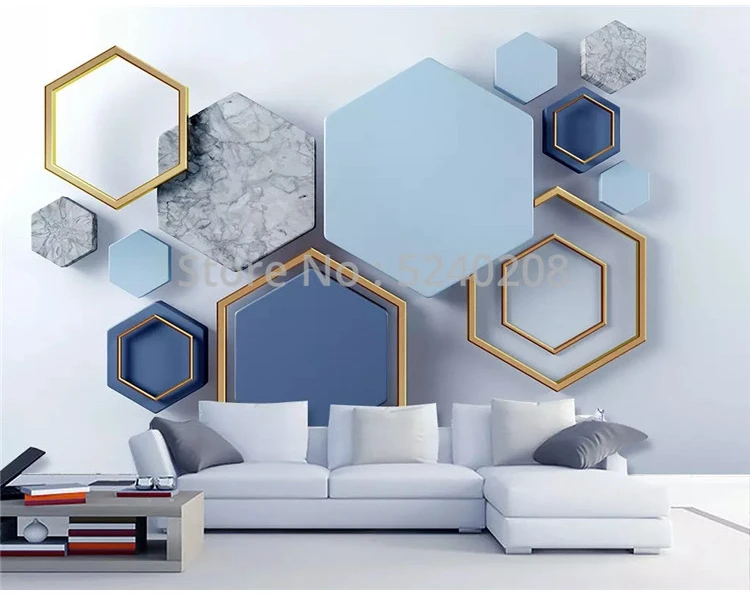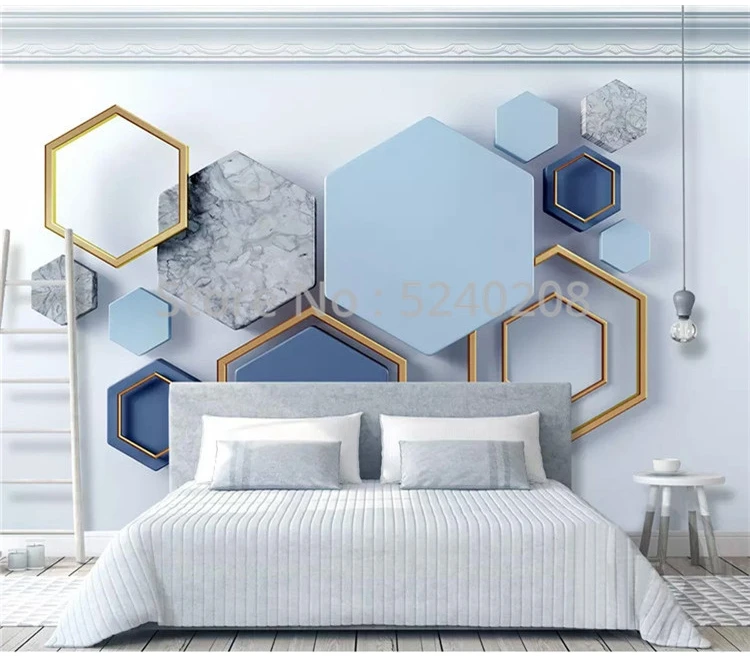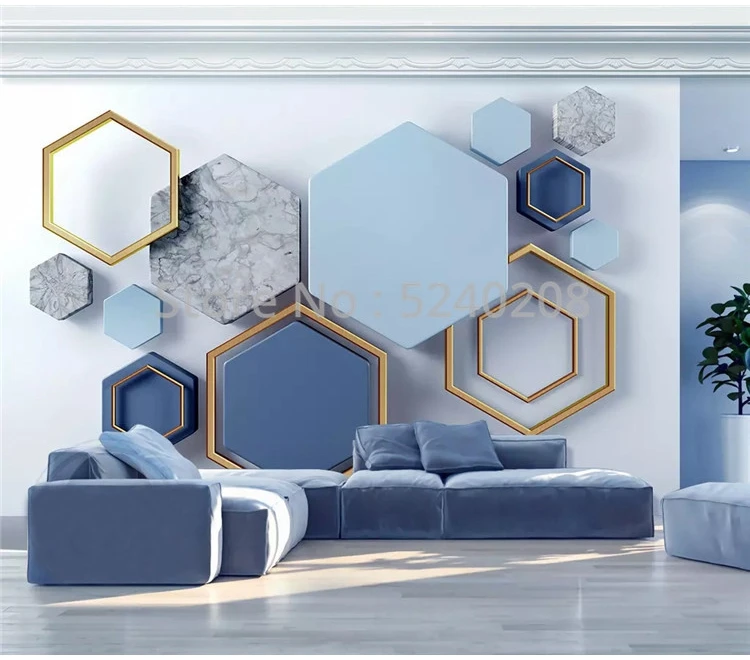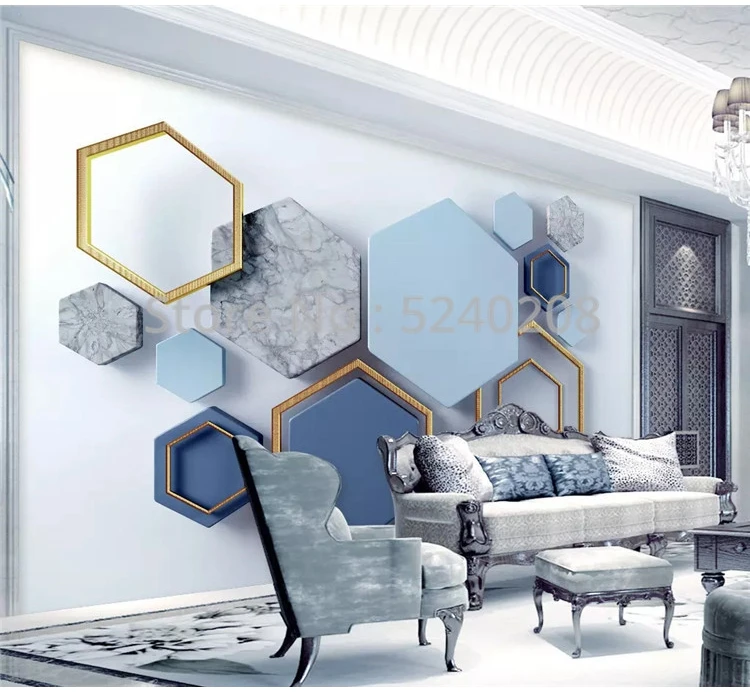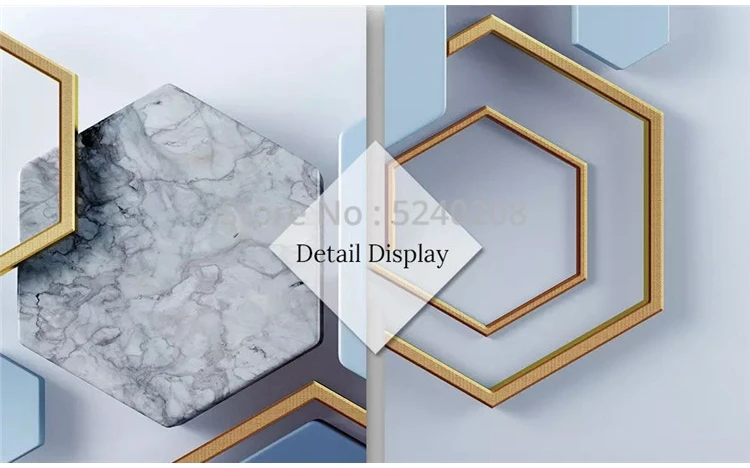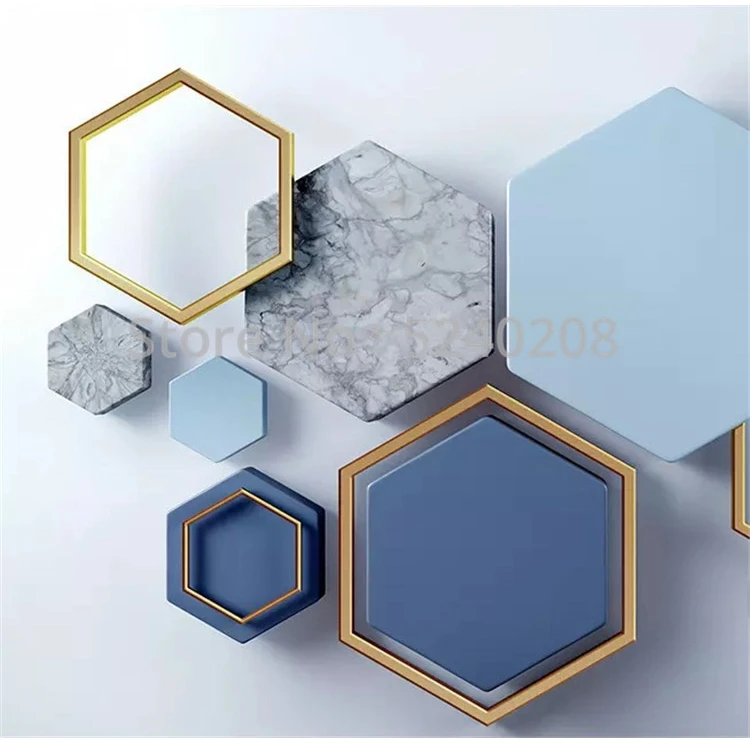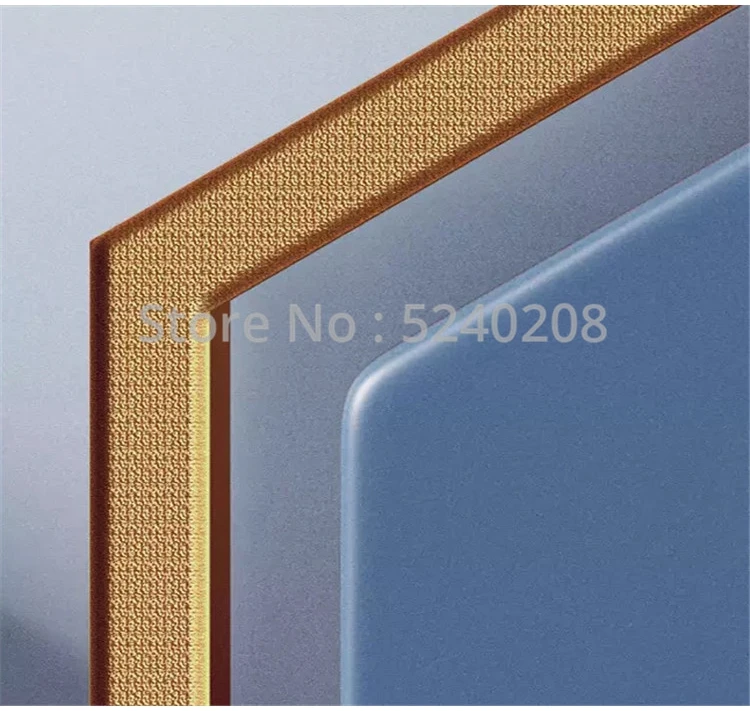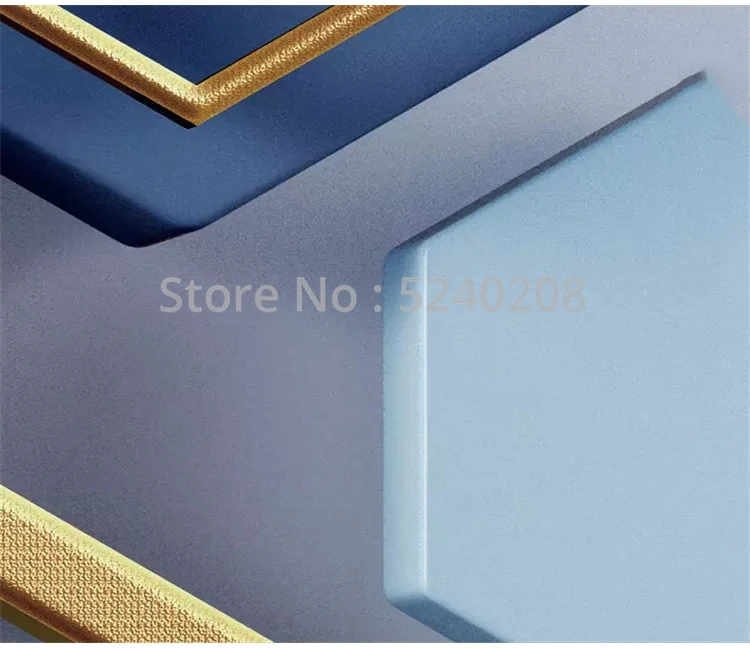Stitching Instructions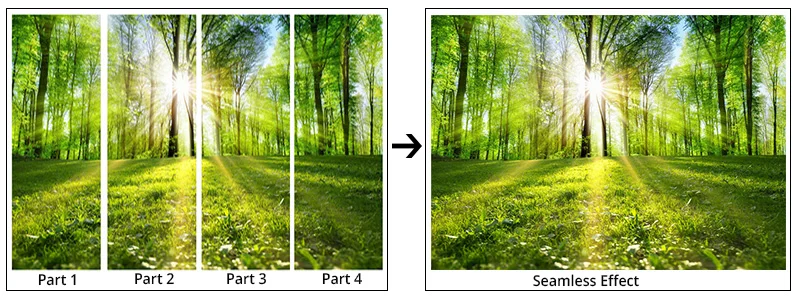▲Tips :Just match the pattern and paste it in order.

Installation Steps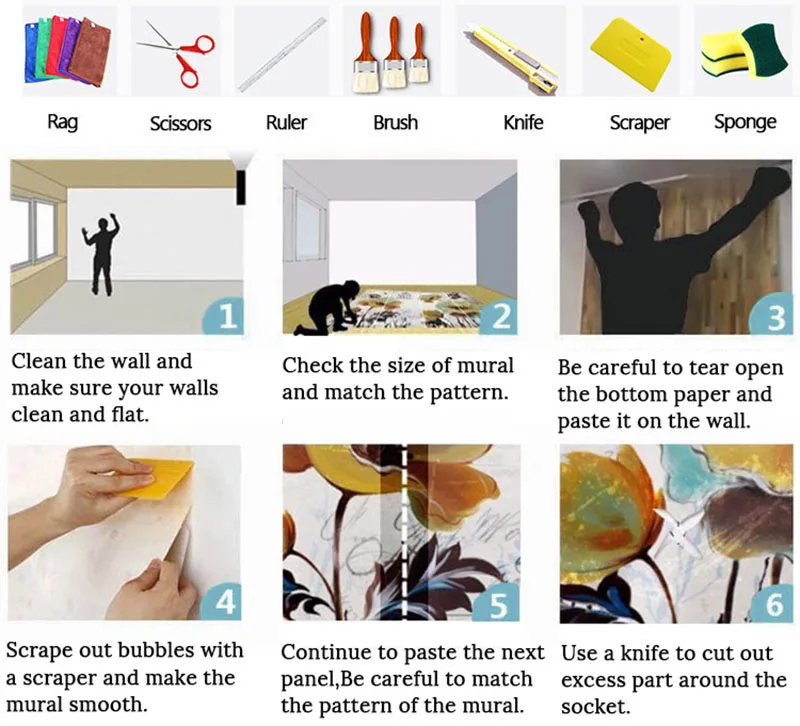Click to edit the product in Ali Orders.
Note: only show for shop owner